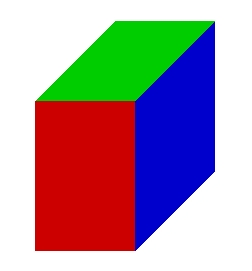# (calculation 22483

The box has the shape of a cuboid with dimensions of 5 cm and 30 mm. Calculate the box's height if the cuboid's volume is 0.60 dm3. Calculate the surface area of ​​the box. (calculation of height from the volume, calculation of area from the formula, keep the same units)

c =  40 cm
S =  670 cm2

### Step-by-step explanation:Did you find an error or inaccuracy? Feel free to write us. Thank you!

Tips for related online calculators
Do you know the volume and unit volume, and want to convert volume units?